Motion in Two and Three Dimensions

# Projectile Motion

### Learning Objectives

By the end of this section, you will be able to:

• Use one-dimensional motion in perpendicular directions to analyze projectile motion.
• Calculate the range, time of flight, and maximum height of a projectile that is launched and impacts a flat, horizontal surface.
• Find the time of flight and impact velocity of a projectile that lands at a different height from that of launch.
• Calculate the trajectory of a projectile.

Projectile motion is the motion of an object thrown or projected into the air, subject only to acceleration as a result of gravity. The applications of projectile motion in physics and engineering are numerous. Some examples include meteors as they enter Earth’s atmosphere, fireworks, and the motion of any ball in sports. Such objects are called projectiles and their path is called a trajectory. The motion of falling objects as discussed in Motion Along a Straight Line is a simple one-dimensional type of projectile motion in which there is no horizontal movement. In this section, we consider two-dimensional projectile motion, and our treatment neglects the effects of air resistance.

The most important fact to remember here is that motions along perpendicular axes are independent and thus can be analyzed separately. We discussed this fact in Displacement and Velocity Vectors, where we saw that vertical and horizontal motions are independent. The key to analyzing two-dimensional projectile motion is to break it into two motions: one along the horizontal axis and the other along the vertical. (This choice of axes is the most sensible because acceleration resulting from gravity is vertical; thus, there is no acceleration along the horizontal axis when air resistance is negligible.) As is customary, we call the horizontal axis the x-axis and the vertical axis the y-axis. It is not required that we use this choice of axes; it is simply convenient in the case of gravitational acceleration. In other cases we may choose a different set of axes. (Figure) illustrates the notation for displacement, where we define $\stackrel{\to }{s}$ to be the total displacement, and $\stackrel{\to }{x}$ and $\stackrel{\to }{y}$ are its component vectors along the horizontal and vertical axes, respectively. The magnitudes of these vectors are s, x, and y.

The total displacement s of a soccer ball at a point along its path. The vector $\stackrel{\to }{s}$ has components $\stackrel{\to }{x}$ and $\stackrel{\to }{y}$ along the horizontal and vertical axes. Its magnitude is s and it makes an angle θ with the horizontal.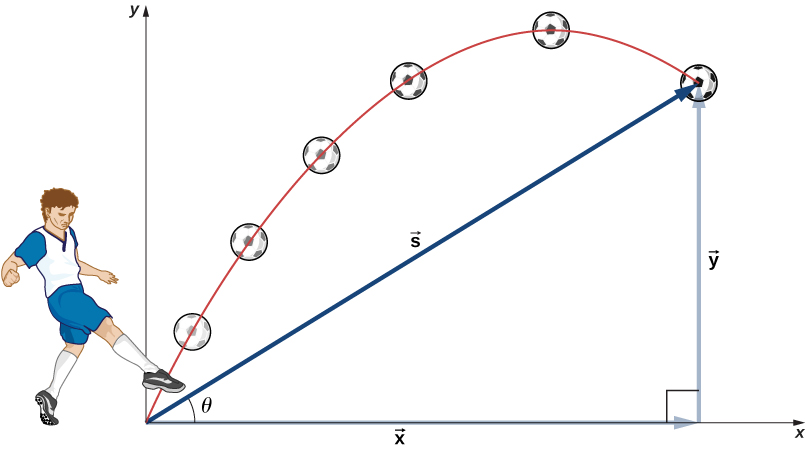To describe projectile motion completely, we must include velocity and acceleration, as well as displacement. We must find their components along the x- and y-axes. Let’s assume all forces except gravity (such as air resistance and friction, for example) are negligible. Defining the positive direction to be upward, the components of acceleration are then very simple:

${a}_{y}=\text{−}g=-9.8\phantom{\rule{0.2em}{0ex}}\text{m}\text{/}{\text{s}}^{2}\phantom{\rule{0.5em}{0ex}}\left(-32\phantom{\rule{0.2em}{0ex}}\text{ft}\text{/}{\text{s}}^{2}\right).$

Because gravity is vertical, ${a}_{x}=0.$ If ${a}_{x}=0,$ this means the initial velocity in the x direction is equal to the final velocity in the x direction, or ${v}_{x}={v}_{0x}.$ With these conditions on acceleration and velocity, we can write the kinematic (Figure) through (Figure) for motion in a uniform gravitational field, including the rest of the kinematic equations for a constant acceleration from Motion with Constant Acceleration. The kinematic equations for motion in a uniform gravitational field become kinematic equations with ${a}_{y}=\text{−}g,\phantom{\rule{0.5em}{0ex}}{a}_{x}=0:$

Horizontal Motion

${v}_{0x}={v}_{x},\phantom{\rule{0.2em}{0ex}}x={x}_{0}+{v}_{x}t$

Vertical Motion

$y={y}_{0}+\frac{1}{2}\left({v}_{0y}+{v}_{y}\right)t$
${v}_{y}={v}_{0y}-gt$
$y={y}_{0}+{v}_{0y}t-\frac{1}{2}g{t}^{2}$
${v}_{y}^{2}={v}_{0y}^{2}-2g\left(y-{y}_{0}\right)$

Using this set of equations, we can analyze projectile motion, keeping in mind some important points.

Problem-Solving Strategy: Projectile Motion
1. Resolve the motion into horizontal and vertical components along the x– and y-axes. The magnitudes of the components of displacement $\stackrel{\to }{s}$ along these axes are x and y. The magnitudes of the components of velocity $\stackrel{\to }{v}$ are ${v}_{x}=v\text{cos}\phantom{\rule{0.1em}{0ex}}\theta \phantom{\rule{0.2em}{0ex}}\text{and}\phantom{\rule{0.2em}{0ex}}{v}_{y}=v\text{sin}\phantom{\rule{0.1em}{0ex}}\theta ,$ where v is the magnitude of the velocity and θ is its direction relative to the horizontal, as shown in (Figure).
2. Treat the motion as two independent one-dimensional motions: one horizontal and the other vertical. Use the kinematic equations for horizontal and vertical motion presented earlier.
3. Solve for the unknowns in the two separate motions: one horizontal and one vertical. Note that the only common variable between the motions is time t. The problem-solving procedures here are the same as those for one-dimensional kinematics and are illustrated in the following solved examples.
4. Recombine quantities in the horizontal and vertical directions to find the total displacement $\stackrel{\to }{s}$ and velocity $\stackrel{\to }{v}.$ Solve for the magnitude and direction of the displacement and velocity using
$s=\sqrt{{x}^{2}+{y}^{2}},\phantom{\rule{0.5em}{0ex}}\theta ={\text{tan}}^{-1}\left(y\text{/}x\right),\phantom{\rule{0.5em}{0ex}}v=\sqrt{{v}_{x}^{2}+{v}_{y}^{2}},$

where θ is the direction of the displacement $\stackrel{\to }{s}.$

(a) We analyze two-dimensional projectile motion by breaking it into two independent one-dimensional motions along the vertical and horizontal axes. (b) The horizontal motion is simple, because ${a}_{x}=0$ and ${v}_{x}$ is a constant. (c) The velocity in the vertical direction begins to decrease as the object rises. At its highest point, the vertical velocity is zero. As the object falls toward Earth again, the vertical velocity increases again in magnitude but points in the opposite direction to the initial vertical velocity. (d) The x and y motions are recombined to give the total velocity at any given point on the trajectory.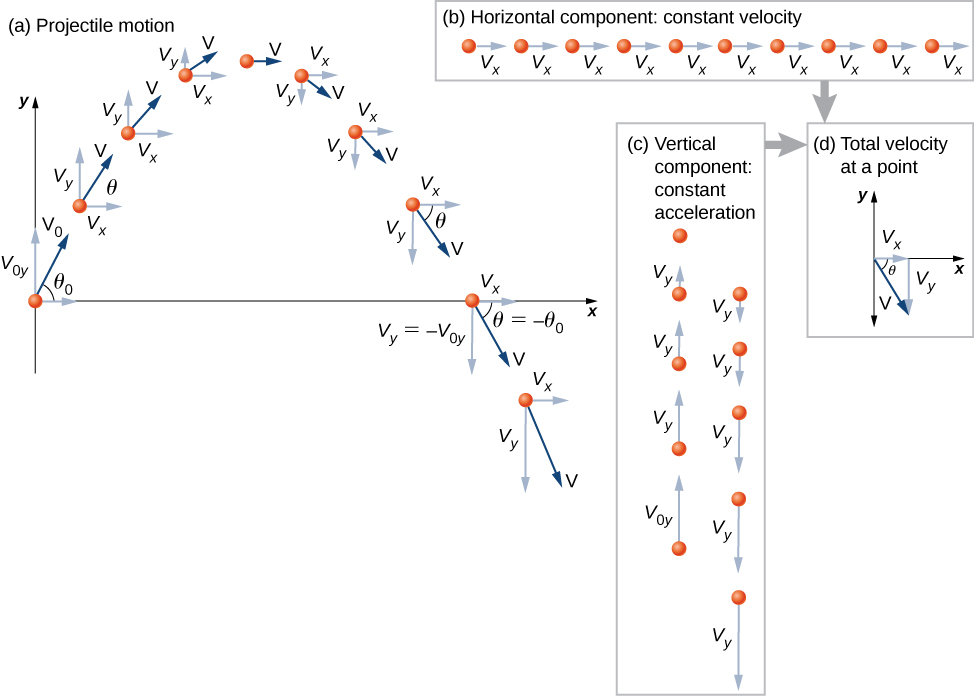A Fireworks Projectile Explodes High and Away
During a fireworks display, a shell is shot into the air with an initial speed of 70.0 m/s at an angle of $75.0\text{°}$ above the horizontal, as illustrated in (Figure). The fuse is timed to ignite the shell just as it reaches its highest point above the ground. (a) Calculate the height at which the shell explodes. (b) How much time passes between the launch of the shell and the explosion? (c) What is the horizontal displacement of the shell when it explodes? (d) What is the total displacement from the point of launch to the highest point?

The trajectory of a fireworks shell. The fuse is set to explode the shell at the highest point in its trajectory, which is found to be at a height of 233 m and 125 m away horizontally.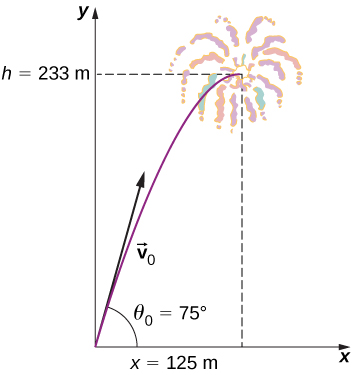Strategy
The motion can be broken into horizontal and vertical motions in which ${a}_{x}=0$ and ${a}_{y}=\text{−}g.$ We can then define ${x}_{0}$ and ${y}_{0}$ to be zero and solve for the desired quantities.

Solution
(a) By “height” we mean the altitude or vertical position y above the starting point. The highest point in any trajectory, called the apex, is reached when ${v}_{y}=0.$ Since we know the initial and final velocities, as well as the initial position, we use the following equation to find y:

${v}_{y}^{2}={v}_{0y}^{2}-2g\left(y-{y}_{0}\right).$

Because ${y}_{0}$ and ${v}_{y}$ are both zero, the equation simplifies to

$\text{0}={v}_{0y}^{2}-2gy.$

Solving for y gives

$y=\frac{{v}_{0y}^{2}}{2g}.$

Now we must find ${v}_{0y},$ the component of the initial velocity in the y direction. It is given by ${v}_{0y}={v}_{0}\text{sin}{\theta }_{0},$ where ${v}_{0}$ is the initial velocity of 70.0 m/s and ${\theta }_{0}=75\text{°}$ is the initial angle. Thus,

${v}_{0y}={v}_{0}\text{sin}\phantom{\rule{0.1em}{0ex}}\theta =\left(70.0\phantom{\rule{0.2em}{0ex}}\text{m}\text{/}\text{s}\right)\text{sin}\phantom{\rule{0.2em}{0ex}}75\text{°}=67.6\phantom{\rule{0.2em}{0ex}}\text{m}\text{/}\text{s}$

and y is

$y=\frac{{\left(67.6\phantom{\rule{0.2em}{0ex}}\text{m}\text{/}\text{s}\right)}^{2}}{2\left(9.80\phantom{\rule{0.2em}{0ex}}\text{m}\text{/}{\text{s}}^{2}\right)}.$

Thus, we have

$y=233\phantom{\rule{0.2em}{0ex}}\text{m}\text{.}$

Note that because up is positive, the initial vertical velocity is positive, as is the maximum height, but the acceleration resulting from gravity is negative. Note also that the maximum height depends only on the vertical component of the initial velocity, so that any projectile with a 67.6-m/s initial vertical component of velocity reaches a maximum height of 233 m (neglecting air resistance). The numbers in this example are reasonable for large fireworks displays, the shells of which do reach such heights before exploding. In practice, air resistance is not completely negligible, so the initial velocity would have to be somewhat larger than that given to reach the same height.

(b) As in many physics problems, there is more than one way to solve for the time the projectile reaches its highest point. In this case, the easiest method is to use ${v}_{y}={v}_{0y}-gt.$ Because ${v}_{y}=0$ at the apex, this equation reduces to simply

$0={v}_{0y}-gt$

or

$t=\frac{{v}_{0y}}{g}=\frac{67.6\phantom{\rule{0.2em}{0ex}}\text{m}\text{/}\text{s}}{9.80\phantom{\rule{0.2em}{0ex}}\text{m}\text{/}{\text{s}}^{2}}=6.90\text{s}\text{.}$

This time is also reasonable for large fireworks. If you are able to see the launch of fireworks, notice that several seconds pass before the shell explodes. Another way of finding the time is by using$y\phantom{\rule{0.2em}{0ex}}\text{=}\phantom{\rule{0.2em}{0ex}}{y}_{0}+\frac{1}{2}\left({v}_{0y}+{v}_{y}\right)t.$ This is left for you as an exercise to complete.

(c) Because air resistance is negligible, ${a}_{x}=0$ and the horizontal velocity is constant, as discussed earlier. The horizontal displacement is the horizontal velocity multiplied by time as given by $x={x}_{0}+{v}_{x}t,$ where ${x}_{0}$ is equal to zero. Thus,

$x={v}_{x}t,$

where ${v}_{x}$ is the x-component of the velocity, which is given by

${v}_{x}={v}_{0}\text{cos}\phantom{\rule{0.1em}{0ex}}\theta =\left(70.0\phantom{\rule{0.2em}{0ex}}\text{m}\text{/}\text{s}\right)\text{cos}75\text{°}=18.1\phantom{\rule{0.2em}{0ex}}\text{m}\text{/}\text{s}.$

Time t for both motions is the same, so x is

$x=\left(18.1\phantom{\rule{0.2em}{0ex}}\text{m}\text{/}\text{s}\right)6.90\phantom{\rule{0.2em}{0ex}}\text{s}=125\phantom{\rule{0.2em}{0ex}}\text{m}\text{.}$

Horizontal motion is a constant velocity in the absence of air resistance. The horizontal displacement found here could be useful in keeping the fireworks fragments from falling on spectators. When the shell explodes, air resistance has a major effect, and many fragments land directly below.

(d) The horizontal and vertical components of the displacement were just calculated, so all that is needed here is to find the magnitude and direction of the displacement at the highest point:

$\stackrel{\to }{s}=125\stackrel{^}{i}+233\stackrel{^}{j}$
$|\stackrel{\to }{s}|=\sqrt{{125}^{2}+{233}^{2}}=264\phantom{\rule{0.2em}{0ex}}\text{m}$
$\theta ={\text{tan}}^{-1}\left(\frac{233}{125}\right)=61.8\text{°}.$

Note that the angle for the displacement vector is less than the initial angle of launch. To see why this is, review (Figure), which shows the curvature of the trajectory toward the ground level.

When solving (Figure)(a), the expression we found for y is valid for any projectile motion when air resistance is negligible. Call the maximum height y = h. Then,

$h=\frac{{v}_{0y}^{2}}{2g}.$

This equation defines the maximum height of a projectile above its launch position and it depends only on the vertical component of the initial velocity.

Check Your Understanding A rock is thrown horizontally off a cliff $100.0\phantom{\rule{0.2em}{0ex}}\text{m}$ high with a velocity of 15.0 m/s. (a) Define the origin of the coordinate system. (b) Which equation describes the horizontal motion? (c) Which equations describe the vertical motion? (d) What is the rock’s velocity at the point of impact?

(a) Choose the top of the cliff where the rock is thrown from the origin of the coordinate system. Although it is arbitrary, we typically choose time t = 0 to correspond to the origin. (b) The equation that describes the horizontal motion is $x={x}_{0}+{v}_{x}t.$ With ${x}_{0}=0,$ this equation becomes $x={v}_{x}t.$ (c) (Figure) through (Figure) and (Figure) describe the vertical motion, but since ${y}_{0}=0\phantom{\rule{0.2em}{0ex}}\text{and}\phantom{\rule{0.2em}{0ex}}{v}_{0y}=0,$ these equations simplify greatly to become $y=\frac{1}{2}\left({v}_{0y}+{v}_{y}\right)t=\frac{1}{2}{v}_{y}t,\phantom{\rule{0.5em}{0ex}}$${v}_{y}=\text{−}gt,\phantom{\rule{0.5em}{0ex}}$$y=-\frac{1}{2}g{t}^{2},\phantom{\rule{0.5em}{0ex}}$ and ${v}_{y}^{2}=-2gy.$ (d) We use the kinematic equations to find the x and y components of the velocity at the point of impact. Using ${v}_{y}^{2}=-2gy$ and noting the point of impact is −100.0 m, we find the y component of the velocity at impact is ${v}_{y}=44.3\phantom{\rule{0.2em}{0ex}}\text{m}\text{/}\text{s}.$ We are given the x component, ${v}_{x}=15.0\phantom{\rule{0.2em}{0ex}}\text{m}\text{/}\text{s},$ so we can calculate the total velocity at impact: v = 46.8 m/s and $\theta =71.3\text{°}$ below the horizontal.

Calculating Projectile Motion: Tennis Player
A tennis player wins a match at Arthur Ashe stadium and hits a ball into the stands at 30 m/s and at an angle $45\text{°}$ above the horizontal ((Figure)). On its way down, the ball is caught by a spectator 10 m above the point where the ball was hit. (a) Calculate the time it takes the tennis ball to reach the spectator. (b) What are the magnitude and direction of the ball’s velocity at impact?

The trajectory of a tennis ball hit into the stands.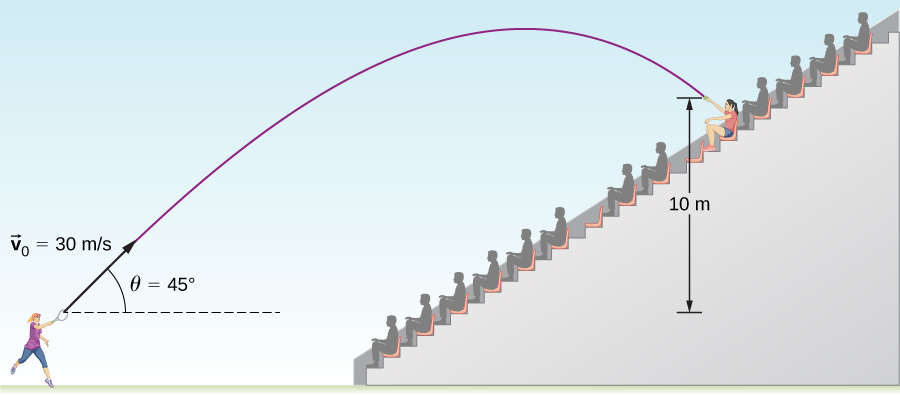Strategy
Again, resolving this two-dimensional motion into two independent one-dimensional motions allows us to solve for the desired quantities. The time a projectile is in the air is governed by its vertical motion alone. Thus, we solve for t first. While the ball is rising and falling vertically, the horizontal motion continues at a constant velocity. This example asks for the final velocity. Thus, we recombine the vertical and horizontal results to obtain $\stackrel{\to }{v}$ at final time t, determined in the first part of the example.

Solution
(a) While the ball is in the air, it rises and then falls to a final position 10.0 m higher than its starting altitude. We can find the time for this by using (Figure):

$y\phantom{\rule{0.2em}{0ex}}\text{=}\phantom{\rule{0.2em}{0ex}}{y}_{0}\phantom{\rule{0.2em}{0ex}}\text{+}\phantom{\rule{0.2em}{0ex}}{v}_{0y}t-\frac{1}{2}g{t}^{2}.$

If we take the initial position ${y}_{0}$ to be zero, then the final position is y = 10 m. The initial vertical velocity is the vertical component of the initial velocity:

${v}_{0y}={v}_{0}\text{sin}\phantom{\rule{0.1em}{0ex}}{\theta }_{0}=\left(30.0\phantom{\rule{0.2em}{0ex}}\text{m}\text{/}\text{s}\right)\text{sin}\phantom{\rule{0.2em}{0ex}}45\text{°}=21.2\phantom{\rule{0.2em}{0ex}}\text{m}\text{/}\text{s}.$

Substituting into (Figure) for y gives us

$10.0\phantom{\rule{0.2em}{0ex}}\text{m}=\left(21.2\phantom{\rule{0.2em}{0ex}}\text{m/s}\right)t-\left(4.90\phantom{\rule{0.2em}{0ex}}{\text{m/s}}^{\text{2}}\right){t}^{2}.$

Rearranging terms gives a quadratic equation in t:

$\left(4.90\phantom{\rule{0.2em}{0ex}}{\text{m/s}}^{\text{2}}\right){t}^{2}-\left(21.2\phantom{\rule{0.2em}{0ex}}\text{m/s}\right)t+10.0\phantom{\rule{0.2em}{0ex}}\text{m}=0.$

Use of the quadratic formula yields t = 3.79 s and t = 0.54 s. Since the ball is at a height of 10 m at two times during its trajectory—once on the way up and once on the way down—we take the longer solution for the time it takes the ball to reach the spectator:

$t=3.79\phantom{\rule{0.2em}{0ex}}\text{s}\text{.}$

The time for projectile motion is determined completely by the vertical motion. Thus, any projectile that has an initial vertical velocity of 21.2 m/s and lands 10.0 m below its starting altitude spends 3.79 s in the air.

(b) We can find the final horizontal and vertical velocities ${v}_{x}$ and ${v}_{y}$ with the use of the result from (a). Then, we can combine them to find the magnitude of the total velocity vector $\stackrel{\to }{v}$ and the angle $\theta$ it makes with the horizontal. Since ${v}_{x}$ is constant, we can solve for it at any horizontal location. We choose the starting point because we know both the initial velocity and the initial angle. Therefore,

${v}_{x}={v}_{0}\text{cos}{\theta }_{0}=\left(30\phantom{\rule{0.2em}{0ex}}\text{m}\text{/}\text{s}\right)\text{cos}\phantom{\rule{0.2em}{0ex}}45\text{°}=21.2\phantom{\rule{0.2em}{0ex}}\text{m}\text{/}\text{s}.$

The final vertical velocity is given by (Figure):

${v}_{y}={v}_{0y}-gt.$

Since ${v}_{0y}$ was found in part (a) to be 21.2 m/s, we have

${v}_{y}=21.2\phantom{\rule{0.2em}{0ex}}\text{m}\text{/}\text{s}-9.8\phantom{\rule{0.2em}{0ex}}\text{m}\text{/}{\text{s}}^{2}\left(3.79\phantom{\rule{0.2em}{0ex}}\text{s}\right)=-15.9\phantom{\rule{0.2em}{0ex}}\text{m}\text{/}\text{s}.$

The magnitude of the final velocity $\stackrel{\to }{v}$ is

$v=\sqrt{{v}_{x}^{2}+{v}_{y}^{2}}=\sqrt{{\left(21.2\phantom{\rule{0.2em}{0ex}}\text{m}\text{/}\text{s}\right)}^{2}+{\left(\text{−}\phantom{\rule{0.2em}{0ex}}\text{15}.9\phantom{\rule{0.2em}{0ex}}\text{m}\text{/}\text{s}\right)}^{2}}=26.5\phantom{\rule{0.2em}{0ex}}\text{m}\text{/}\text{s}.$

The direction ${\theta }_{v}$ is found using the inverse tangent:

${\theta }_{v}={\text{tan}}^{-1}\left(\frac{{v}_{y}}{{v}_{x}}\right)={\text{tan}}^{-1}\left(\frac{21.2}{-15.9}\right)=-53.1\text{°}.$

Significance
(a) As mentioned earlier, the time for projectile motion is determined completely by the vertical motion. Thus, any projectile that has an initial vertical velocity of 21.2 m/s and lands 10.0 m below its starting altitude spends 3.79 s in the air. (b) The negative angle means the velocity is $53.1\text{°}$ below the horizontal at the point of impact. This result is consistent with the fact that the ball is impacting at a point on the other side of the apex of the trajectory and therefore has a negative y component of the velocity. The magnitude of the velocity is less than the magnitude of the initial velocity we expect since it is impacting 10.0 m above the launch elevation.

### Time of Flight, Trajectory, and Range

Of interest are the time of flight, trajectory, and range for a projectile launched on a flat horizontal surface and impacting on the same surface. In this case, kinematic equations give useful expressions for these quantities, which are derived in the following sections.

#### Time of flight

We can solve for the time of flight of a projectile that is both launched and impacts on a flat horizontal surface by performing some manipulations of the kinematic equations. We note the position and displacement in y must be zero at launch and at impact on an even surface. Thus, we set the displacement in y equal to zero and find

$y-{y}_{0}={v}_{0y}t-\frac{1}{2}g{t}^{2}=\left({v}_{0}\text{sin}{\theta }_{0}\right)t-\frac{1}{2}g{t}^{2}=0.$

Factoring, we have

$t\left({v}_{0}\text{sin}{\theta }_{0}-\frac{gt}{2}\right)=0.$

Solving for t gives us

${T}_{\text{tof}}=\frac{2\left({v}_{0}\text{sin}{\theta }_{0}\right)}{g}.$

This is the time of flight for a projectile both launched and impacting on a flat horizontal surface. (Figure) does not apply when the projectile lands at a different elevation than it was launched, as we saw in (Figure) of the tennis player hitting the ball into the stands. The other solution, t = 0, corresponds to the time at launch. The time of flight is linearly proportional to the initial velocity in the y direction and inversely proportional to g. Thus, on the Moon, where gravity is one-sixth that of Earth, a projectile launched with the same velocity as on Earth would be airborne six times as long.

#### Trajectory

The trajectory of a projectile can be found by eliminating the time variable t from the kinematic equations for arbitrary t and solving for y(x). We take ${x}_{0}={y}_{0}=0$ so the projectile is launched from the origin. The kinematic equation for x gives

$x={v}_{0x}t⇒t=\frac{x}{{v}_{0x}}=\frac{x}{{v}_{0}\text{cos}{\theta }_{0}}.$

Substituting the expression for t into the equation for the position $y=\left({v}_{0}\text{sin}{\theta }_{0}\right)t-\frac{1}{2}g{t}^{2}$ gives

$y=\left({v}_{0}\text{sin}\phantom{\rule{0.1em}{0ex}}{\theta }_{0}\right)\left(\frac{x}{{v}_{0}\text{cos}\phantom{\rule{0.1em}{0ex}}{\theta }_{0}}\right)-\frac{1}{2}g{\left(\frac{x}{{v}_{0}\text{cos}\phantom{\rule{0.1em}{0ex}}{\theta }_{0}}\right)}^{2}.$

Rearranging terms, we have

$y=\left(\text{tan}\phantom{\rule{0.1em}{0ex}}{\theta }_{0}\right)x-\left[\frac{g}{2{\left({v}_{0}\text{cos}\phantom{\rule{0.1em}{0ex}}{\theta }_{0}\right)}^{2}}\right]{x}^{2}.$

This trajectory equation is of the form $y=ax+b{x}^{2},$ which is an equation of a parabola with coefficients

$a=\text{tan}\phantom{\rule{0.1em}{0ex}}{\theta }_{0},\phantom{\rule{0.5em}{0ex}}b=-\frac{g}{2{\left({v}_{0}\text{cos}\phantom{\rule{0.1em}{0ex}}{\theta }_{0}\right)}^{2}}.$

#### Range

From the trajectory equation we can also find the range, or the horizontal distance traveled by the projectile. Factoring (Figure), we have

$y=x\left[\text{tan}\phantom{\rule{0.1em}{0ex}}{\theta }_{0}-\frac{g}{2{\left({v}_{0}\text{cos}\phantom{\rule{0.1em}{0ex}}{\theta }_{0}\right)}^{2}}x\right].$

The position y is zero for both the launch point and the impact point, since we are again considering only a flat horizontal surface. Setting y = 0 in this equation gives solutions x = 0, corresponding to the launch point, and

$x=\frac{2{v}_{0}^{2}\text{sin}\phantom{\rule{0.1em}{0ex}}{\theta }_{0}\text{cos}\phantom{\rule{0.1em}{0ex}}{\theta }_{0}}{g},$

corresponding to the impact point. Using the trigonometric identity $2\text{sin}\phantom{\rule{0.1em}{0ex}}\theta \text{cos}\phantom{\rule{0.1em}{0ex}}\theta =\text{sin}2\theta$ and setting x = R for range, we find

$R=\frac{{v}_{0}^{2}\text{sin}2{\theta }_{0}}{g}.$

Note particularly that (Figure) is valid only for launch and impact on a horizontal surface. We see the range is directly proportional to the square of the initial speed ${v}_{0}$ and $\text{sin}2{\theta }_{0}$, and it is inversely proportional to the acceleration of gravity. Thus, on the Moon, the range would be six times greater than on Earth for the same initial velocity. Furthermore, we see from the factor $\text{sin}2{\theta }_{0}$ that the range is maximum at $45\text{°}.$ These results are shown in (Figure). In (a) we see that the greater the initial velocity, the greater the range. In (b), we see that the range is maximum at $45\text{°}.$ This is true only for conditions neglecting air resistance. If air resistance is considered, the maximum angle is somewhat smaller. It is interesting that the same range is found for two initial launch angles that sum to $90\text{°}.$ The projectile launched with the smaller angle has a lower apex than the higher angle, but they both have the same range.

Trajectories of projectiles on level ground. (a) The greater the initial speed ${v}_{0},$ the greater the range for a given initial angle. (b) The effect of initial angle ${\theta }_{0}$ on the range of a projectile with a given initial speed. Note that the range is the same for initial angles of $15\text{°}$ and $75\text{°},$ although the maximum heights of those paths are different.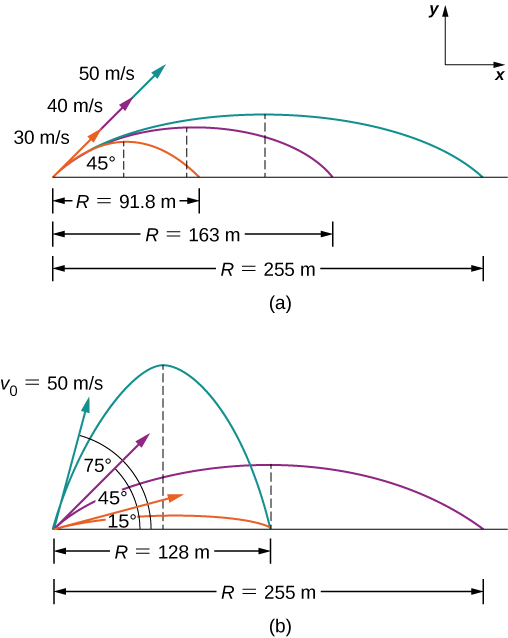Comparing Golf Shots
A golfer finds himself in two different situations on different holes. On the second hole he is 120 m from the green and wants to hit the ball 90 m and let it run onto the green. He angles the shot low to the ground at $30\text{°}$ to the horizontal to let the ball roll after impact. On the fourth hole he is 90 m from the green and wants to let the ball drop with a minimum amount of rolling after impact. Here, he angles the shot at $70\text{°}$ to the horizontal to minimize rolling after impact. Both shots are hit and impacted on a level surface.

(a) What is the initial speed of the ball at the second hole?

(b) What is the initial speed of the ball at the fourth hole?

(c) Write the trajectory equation for both cases.

(d) Graph the trajectories.

Strategy
We see that the range equation has the initial speed and angle, so we can solve for the initial speed for both (a) and (b). When we have the initial speed, we can use this value to write the trajectory equation.

Solution
(a) $R=\frac{{v}_{0}^{2}\text{sin}\phantom{\rule{0.1em}{0ex}}2{\theta }_{0}}{g}⇒{v}_{0}=\sqrt{\frac{Rg}{\text{sin}\phantom{\rule{0.1em}{0ex}}2{\theta }_{0}}}=\sqrt{\frac{90.0\phantom{\rule{0.2em}{0ex}}\text{m}\left(9.8\phantom{\rule{0.2em}{0ex}}\text{m}\text{/}{\text{s}}^{2}\right)}{\text{sin}\left(2\left(70\text{°}\right)\right)}}=37.0\phantom{\rule{0.2em}{0ex}}\text{m}\text{/}\text{s}$

(b) $R=\frac{{v}_{0}^{2}\text{sin}\phantom{\rule{0.1em}{0ex}}2{\theta }_{0}}{g}⇒{v}_{0}=\sqrt{\frac{Rg}{\text{sin}\phantom{\rule{0.1em}{0ex}}2{\theta }_{0}}}=\sqrt{\frac{90.0\phantom{\rule{0.2em}{0ex}}\text{m}\left(9.8\phantom{\rule{0.2em}{0ex}}\text{m}\text{/}{\text{s}}^{2}\right)}{\text{sin}\left(2\left(30\text{°}\right)\right)}}=31.9\phantom{\rule{0.2em}{0ex}}\text{m}\text{/}\text{s}$

(c)

$\begin{array}{}\\ \\ \\ y=x\left[\text{tan}\phantom{\rule{0.1em}{0ex}}{\theta }_{0}-\frac{g}{2{\left({v}_{0}\text{cos}\phantom{\rule{0.1em}{0ex}}{\theta }_{0}\right)}^{2}}x\right]\hfill \\ \text{Second hole:}\phantom{\rule{0.2em}{0ex}}y=x\left[\text{tan}\phantom{\rule{0.2em}{0ex}}70\text{°}-\frac{9.8\phantom{\rule{0.2em}{0ex}}\text{m}\text{/}{\text{s}}^{2}}{2{\left[\left(37.0\phantom{\rule{0.2em}{0ex}}\text{m}\text{/}\text{s)(}\text{cos}\phantom{\rule{0.2em}{0ex}}70\text{°}\right)\right]}^{2}}x\right]=2.75x-0.0306{x}^{2}\hfill \\ \text{Fourth hole:}\phantom{\rule{0.2em}{0ex}}y=x\left[\text{tan}\phantom{\rule{0.2em}{0ex}}30\text{°}-\frac{9.8\phantom{\rule{0.2em}{0ex}}\text{m}\text{/}{\text{s}}^{2}}{2{\left[\left(31.9\phantom{\rule{0.2em}{0ex}}\text{m}\text{/}\text{s)(}\text{cos}30\text{°}\right)\right]}^{2}}x\right]=0.58x-0.0064{x}^{2}\hfill \end{array}$

(d) Using a graphing utility, we can compare the two trajectories, which are shown in (Figure).

Two trajectories of a golf ball with a range of 90 m. The impact points of both are at the same level as the launch point.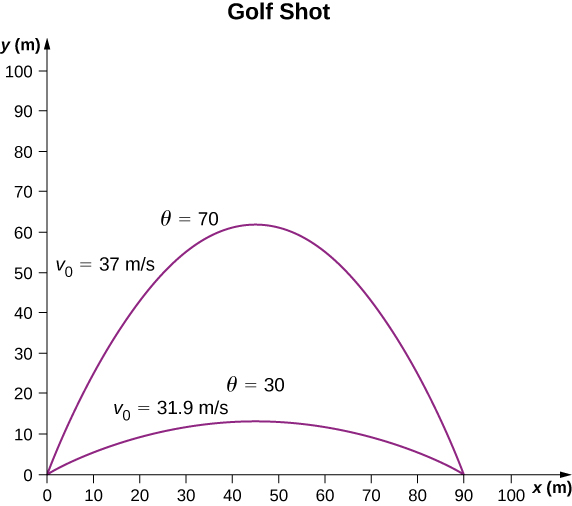Significance
The initial speed for the shot at $70\text{°}$ is greater than the initial speed of the shot at $30\text{°}.$ Note from (Figure) that two projectiles launched at the same speed but at different angles have the same range if the launch angles add to $90\text{°}.$ The launch angles in this example add to give a number greater than $90\text{°}.$ Thus, the shot at $70\text{°}$ has to have a greater launch speed to reach 90 m, otherwise it would land at a shorter distance.

Check Your Understanding If the two golf shots in (Figure) were launched at the same speed, which shot would have the greatest range?

The golf shot at $30\text{°}.$

When we speak of the range of a projectile on level ground, we assume R is very small compared with the circumference of Earth. If, however, the range is large, Earth curves away below the projectile and the acceleration resulting from gravity changes direction along the path. The range is larger than predicted by the range equation given earlier because the projectile has farther to fall than it would on level ground, as shown in (Figure), which is based on a drawing in Newton’s Principia . If the initial speed is great enough, the projectile goes into orbit. Earth’s surface drops 5 m every 8000 m. In 1 s an object falls 5 m without air resistance. Thus, if an object is given a horizontal velocity of $8000\phantom{\rule{0.2em}{0ex}}\text{m}\text{/}\text{s}$ (or $18,000\text{mi}\text{/}\text{hr}\right)$ near Earth’s surface, it will go into orbit around the planet because the surface continuously falls away from the object. This is roughly the speed of the Space Shuttle in a low Earth orbit when it was operational, or any satellite in a low Earth orbit. These and other aspects of orbital motion, such as Earth’s rotation, are covered in greater depth in Gravitation.

Projectile to satellite. In each case shown here, a projectile is launched from a very high tower to avoid air resistance. With increasing initial speed, the range increases and becomes longer than it would be on level ground because Earth curves away beneath its path. With a speed of 8000 m/s, orbit is achieved.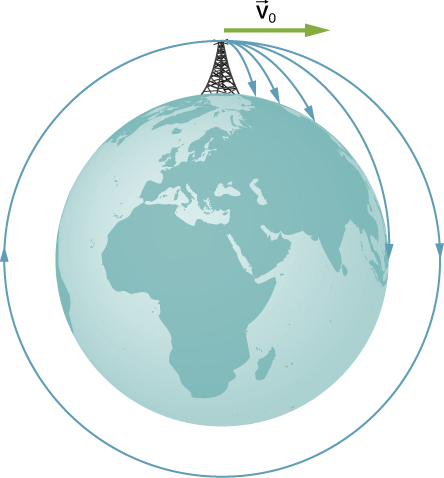At PhET Explorations: Projectile Motion, learn about projectile motion in terms of the launch angle and initial velocity.

### Summary

• Projectile motion is the motion of an object subject only to the acceleration of gravity, where the acceleration is constant, as near the surface of Earth.
• To solve projectile motion problems, we analyze the motion of the projectile in the horizontal and vertical directions using the one-dimensional kinematic equations for x and y.
• The time of flight of a projectile launched with initial vertical velocity ${v}_{0y}$ on an even surface is given by
${T}_{tof}=\frac{2\left({v}_{0}\text{sin}\phantom{\rule{0.1em}{0ex}}\theta \right)}{g}.$

This equation is valid only when the projectile lands at the same elevation from which it was launched.

• The maximum horizontal distance traveled by a projectile is called the range. Again, the equation for range is valid only when the projectile lands at the same elevation from which it was launched.

### Conceptual Questions

Answer the following questions for projectile motion on level ground assuming negligible air resistance, with the initial angle being neither $0\text{°}$ nor $90\text{°}:$ (a) Is the velocity ever zero? (b) When is the velocity a minimum? A maximum? (c) Can the velocity ever be the same as the initial velocity at a time other than at t = 0? (d) Can the speed ever be the same as the initial speed at a time other than at t = 0?

a. no; b. minimum at apex of trajectory and maximum at launch and impact; c. no, velocity is a vector; d. yes, where it lands

Answer the following questions for projectile motion on level ground assuming negligible air resistance, with the initial angle being neither $0\text{°}$ nor $90\text{°}:$ (a) Is the acceleration ever zero? (b) Is the acceleration ever in the same direction as a component of velocity? (c) Is the acceleration ever opposite in direction to a component of velocity?

A dime is placed at the edge of a table so it hangs over slightly. A quarter is slid horizontally on the table surface perpendicular to the edge and hits the dime head on. Which coin hits the ground first?

They both hit the ground at the same time.

### Problems

A bullet is shot horizontally from shoulder height (1.5 m) with and initial speed 200 m/s. (a) How much time elapses before the bullet hits the ground? (b) How far does the bullet travel horizontally?

a. $t=0.55\phantom{\rule{0.2em}{0ex}}\text{s}$, b. $x=110\phantom{\rule{0.2em}{0ex}}\text{m}$

A marble rolls off a tabletop 1.0 m high and hits the floor at a point 3.0 m away from the table’s edge in the horizontal direction. (a) How long is the marble in the air? (b) What is the speed of the marble when it leaves the table’s edge? (c) What is its speed when it hits the floor?

A dart is thrown horizontally at a speed of 10 m/s at the bull’s-eye of a dartboard 2.4 m away, as in the following figure. (a) How far below the intended target does the dart hit? (b) What does your answer tell you about how proficient dart players throw their darts?

a. $t=0.24\text{s,}\phantom{\rule{0.5em}{0ex}}d=0.28\phantom{\rule{0.2em}{0ex}}\text{m}$, b. They aim high.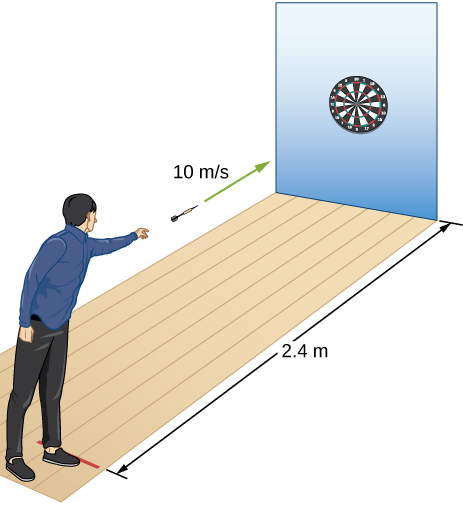An airplane flying horizontally with a speed of 500 km/h at a height of 800 m drops a crate of supplies (see the following figure). If the parachute fails to open, how far in front of the release point does the crate hit the ground?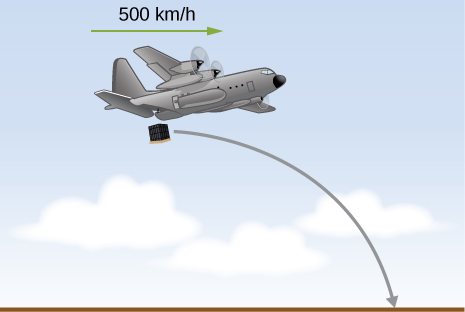Suppose the airplane in the preceding problem fires a projectile horizontally in its direction of motion at a speed of 300 m/s relative to the plane. (a) How far in front of the release point does the projectile hit the ground? (b) What is its speed when it hits the ground?

a., $t=12.8\phantom{\rule{0.2em}{0ex}}\text{s,}\phantom{\rule{0.5em}{0ex}}x=5619\phantom{\rule{0.2em}{0ex}}\text{m}$ b. ${v}_{y}=125.0\phantom{\rule{0.2em}{0ex}}\text{m}\text{/}\text{s,}\phantom{\rule{0.5em}{0ex}}{v}_{x}=439.0\phantom{\rule{0.2em}{0ex}}\text{m}\text{/}\text{s,}\phantom{\rule{0.5em}{0ex}}|\stackrel{\to }{v}|=456.0\phantom{\rule{0.2em}{0ex}}\text{m}\text{/}\text{s}$

A fastball pitcher can throw a baseball at a speed of 40 m/s (90 mi/h). (a) Assuming the pitcher can release the ball 16.7 m from home plate so the ball is moving horizontally, how long does it take the ball to reach home plate? (b) How far does the ball drop between the pitcher’s hand and home plate?

A projectile is launched at an angle of $30\text{°}$ and lands 20 s later at the same height as it was launched. (a) What is the initial speed of the projectile? (b) What is the maximum altitude? (c) What is the range? (d) Calculate the displacement from the point of launch to the position on its trajectory at 15 s.

a. ${v}_{y}={v}_{0y}-gt,\phantom{\rule{0.5em}{0ex}}t=10\text{s,}\phantom{\rule{0.5em}{0ex}}{v}_{y}=0,\phantom{\rule{0.5em}{0ex}}{v}_{0y}=98.0\phantom{\rule{0.2em}{0ex}}\text{m}\text{/}\text{s},\phantom{\rule{0.5em}{0ex}}{v}_{0}=196.0\phantom{\rule{0.2em}{0ex}}\text{m}\text{/}\text{s}$, b. $h=490.0\phantom{\rule{0.2em}{0ex}}\text{m},$

c. ${v}_{0x}=169.7\phantom{\rule{0.2em}{0ex}}\text{m}\text{/}\text{s,}\phantom{\rule{0.5em}{0ex}}x=3394.0\phantom{\rule{0.2em}{0ex}}\text{m,}$

d. $\begin{array}{}\\ \\ x=2545.5\phantom{\rule{0.2em}{0ex}}\text{m}\hfill \\ y=465.5\phantom{\rule{0.2em}{0ex}}\text{m}\hfill \\ \stackrel{\to }{s}=2545.5\phantom{\rule{0.2em}{0ex}}\text{m}\stackrel{^}{i}+465.5\phantom{\rule{0.2em}{0ex}}\text{m}\stackrel{^}{j}\hfill \end{array}$

A basketball player shoots toward a basket 6.1 m away and 3.0 m above the floor. If the ball is released 1.8 m above the floor at an angle of $60\text{°}$ above the horizontal, what must the initial speed be if it were to go through the basket?

At a particular instant, a hot air balloon is 100 m in the air and descending at a constant speed of 2.0 m/s. At this exact instant, a girl throws a ball horizontally, relative to herself, with an initial speed of 20 m/s. When she lands, where will she find the ball? Ignore air resistance.

$-100\phantom{\rule{0.2em}{0ex}}\text{m}=\left(-2.0\phantom{\rule{0.2em}{0ex}}\text{m}\text{/}\text{s}\right)t-\left(4.9\phantom{\rule{0.2em}{0ex}}\text{m}\text{/}{\text{s}}^{2}\right){t}^{2},$$t=4.3\phantom{\rule{0.2em}{0ex}}\text{s,}$$x=86.0\phantom{\rule{0.2em}{0ex}}\text{m}$

A man on a motorcycle traveling at a uniform speed of 10 m/s throws an empty can straight upward relative to himself with an initial speed of 3.0 m/s. Find the equation of the trajectory as seen by a police officer on the side of the road. Assume the initial position of the can is the point where it is thrown. Ignore air resistance.

An athlete can jump a distance of 8.0 m in the broad jump. What is the maximum distance the athlete can jump on the Moon, where the gravitational acceleration is one-sixth that of Earth?

${R}_{Moon}=48\phantom{\rule{0.2em}{0ex}}\text{m}$

The maximum horizontal distance a boy can throw a ball is 50 m. Assume he can throw with the same initial speed at all angles. How high does he throw the ball when he throws it straight upward?

A rock is thrown off a cliff at an angle of $53\text{°}$ with respect to the horizontal. The cliff is 100 m high. The initial speed of the rock is 30 m/s. (a) How high above the edge of the cliff does the rock rise? (b) How far has it moved horizontally when it is at maximum altitude? (c) How long after the release does it hit the ground? (d) What is the range of the rock? (e) What are the horizontal and vertical positions of the rock relative to the edge of the cliff at t = 2.0 s, t = 4.0 s, and t = 6.0 s?

a. ${v}_{0y}=24\phantom{\rule{0.2em}{0ex}}\text{m}\text{/}\text{s}$${v}_{y}^{2}={v}_{0y}^{2}-2gy⇒h=23.4\phantom{\rule{0.2em}{0ex}}\text{m}$,

b. $t=3\phantom{\rule{0.2em}{0ex}}\text{s}\phantom{\rule{0.5em}{0ex}}{v}_{0x}=18\phantom{\rule{0.2em}{0ex}}\text{m/s}\phantom{\rule{0.5em}{0ex}}x=54\phantom{\rule{0.2em}{0ex}}\text{m}$,

c. $y=-100\phantom{\rule{0.2em}{0ex}}\text{m}\phantom{\rule{0.5em}{0ex}}{y}_{0}=0$ $y-{y}_{0}={v}_{0y}t-\frac{1}{2}g{t}^{2}\phantom{\rule{0.5em}{0ex}}-100=24t-4.9{t}^{2}$ $⇒t=7.58\phantom{\rule{0.2em}{0ex}}\text{s}$,

d. $x=136.44\phantom{\rule{0.2em}{0ex}}\text{m}$,

e. $t=2.0\phantom{\rule{0.2em}{0ex}}\text{s}\phantom{\rule{0.5em}{0ex}}y=28.4\phantom{\rule{0.2em}{0ex}}\text{m}\phantom{\rule{0.5em}{0ex}}x=36\phantom{\rule{0.2em}{0ex}}\text{m}$

$t=4.0\phantom{\rule{0.2em}{0ex}}\text{s}\phantom{\rule{0.5em}{0ex}}y=17.6\phantom{\rule{0.2em}{0ex}}\text{m}\phantom{\rule{0.5em}{0ex}}x=22.4\phantom{\rule{0.2em}{0ex}}\text{m}$

$t=6.0\phantom{\rule{0.2em}{0ex}}\text{s}\phantom{\rule{0.5em}{0ex}}y=-32.4\phantom{\rule{0.2em}{0ex}}\text{m}\phantom{\rule{0.5em}{0ex}}x=108\phantom{\rule{0.2em}{0ex}}\text{m}$

Trying to escape his pursuers, a secret agent skis off a slope inclined at $30\text{°}$ below the horizontal at 60 km/h. To survive and land on the snow 100 m below, he must clear a gorge 60 m wide. Does he make it? Ignore air resistance.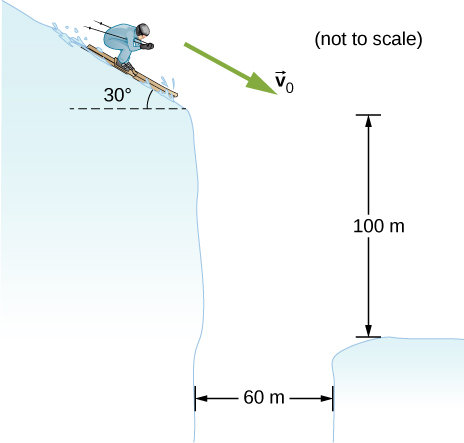A golfer on a fairway is 70 m away from the green, which sits below the level of the fairway by 20 m. If the golfer hits the ball at an angle of $40\text{°}$ with an initial speed of 20 m/s, how close to the green does she come?

${v}_{0y}=12.9\phantom{\rule{0.2em}{0ex}}\text{m}\text{/}\text{s}\phantom{\rule{0.2em}{0ex}}y-{y}_{0}={v}_{0y}t-\frac{1}{2}g{t}^{2}\phantom{\rule{0.5em}{0ex}}-20.0=12.9t-4.9{t}^{2}$

$t=3.7\phantom{\rule{0.2em}{0ex}}\text{s}\phantom{\rule{0.5em}{0ex}}{v}_{0x}=15.3\phantom{\rule{0.2em}{0ex}}\text{m}\text{/}\text{s}⇒x=56.7\phantom{\rule{0.2em}{0ex}}\text{m}$

So the golfer’s shot lands 13.3 m short of the green.

A projectile is shot at a hill, the base of which is 300 m away. The projectile is shot at $60\text{°}$ above the horizontal with an initial speed of 75 m/s. The hill can be approximated by a plane sloped at $20\text{°}$ to the horizontal. Relative to the coordinate system shown in the following figure, the equation of this straight line is $y=\left(\text{tan}20\text{°}\right)x-109.$ Where on the hill does the projectile land?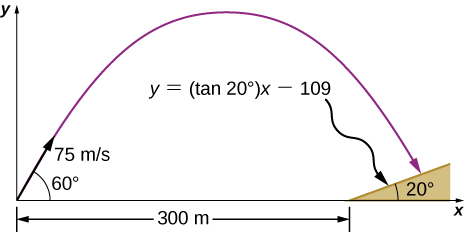An astronaut on Mars kicks a soccer ball at an angle of $45\text{°}$ with an initial velocity of 15 m/s. If the acceleration of gravity on Mars is 3.7 m/s, (a) what is the range of the soccer kick on a flat surface? (b) What would be the range of the same kick on the Moon, where gravity is one-sixth that of Earth?

a. $R=60.8\phantom{\rule{0.2em}{0ex}}\text{m}$,

b. $R=137.8\phantom{\rule{0.2em}{0ex}}\text{m}$

Mike Powell holds the record for the long jump of 8.95 m, established in 1991. If he left the ground at an angle of $15\text{°},$ what was his initial speed?

MIT’s robot cheetah can jump over obstacles 46 cm high and has speed of 12.0 km/h. (a) If the robot launches itself at an angle of $60\text{°}$ at this speed, what is its maximum height? (b) What would the launch angle have to be to reach a height of 46 cm?

a. ${v}_{y}^{2}={v}_{0y}^{2}-2gy⇒y=2.9\phantom{\rule{0.2em}{0ex}}\text{m}\text{/}\text{s}$

$y=3.3\phantom{\rule{0.2em}{0ex}}\text{m}\text{/}\text{s}$

$y=\frac{{v}_{0y}^{2}}{2g}=\frac{{\left({v}_{0}\text{sin}\phantom{\rule{0.1em}{0ex}}\theta \right)}^{2}}{2g}⇒\text{sin}\phantom{\rule{0.1em}{0ex}}\theta =0.91⇒\theta =65.5\text{°}$

Mt. Asama, Japan, is an active volcano. In 2009, an eruption threw solid volcanic rocks that landed 1 km horizontally from the crater. If the volcanic rocks were launched at an angle of $40\text{°}$ with respect to the horizontal and landed 900 m below the crater, (a) what would be their initial velocity and (b) what is their time of flight?

Drew Brees of the New Orleans Saints can throw a football 23.0 m/s (50 mph). If he angles the throw at $10\text{°}$ from the horizontal, what distance does it go if it is to be caught at the same elevation as it was thrown?

$R=18.5\phantom{\rule{0.2em}{0ex}}\text{m}$

The Lunar Roving Vehicle used in NASA’s late Apollo missions reached an unofficial lunar land speed of 5.0 m/s by astronaut Eugene Cernan. If the rover was moving at this speed on a flat lunar surface and hit a small bump that projected it off the surface at an angle of $20\text{°},$ how long would it be “airborne” on the Moon?

A soccer goal is 2.44 m high. A player kicks the ball at a distance 10 m from the goal at an angle of $25\text{°}.$ What is the initial speed of the soccer ball?

$y=\left(\text{tan}\phantom{\rule{0.1em}{0ex}}{\theta }_{0}\right)x-\left[\frac{g}{2{\left({v}_{0}\text{cos}\phantom{\rule{0.1em}{0ex}}{\theta }_{0}\right)}^{2}}\right]{x}^{2}⇒{v}_{0}=16.4\phantom{\rule{0.2em}{0ex}}\text{m}\text{/}\text{s}$

Olympus Mons on Mars is the largest volcano in the solar system, at a height of 25 km and with a radius of 312 km. If you are standing on the summit, with what initial velocity would you have to fire a projectile from a cannon horizontally to clear the volcano and land on the surface of Mars? Note that Mars has an acceleration of gravity of $3.7\phantom{\rule{0.2em}{0ex}}\text{m}\text{/}{\text{s}}^{2}.$

In 1999, Robbie Knievel was the first to jump the Grand Canyon on a motorcycle. At a narrow part of the canyon (69.0 m wide) and traveling 35.8 m/s off the takeoff ramp, he reached the other side. What was his launch angle?

$R=\frac{{v}_{0}^{2}\text{sin}\phantom{\rule{0.2em}{0ex}}2{\theta }_{0}}{g}⇒{\theta }_{0}=15.0\text{°}$

You throw a baseball at an initial speed of 15.0 m/s at an angle of $30\text{°}$ with respect to the horizontal. What would the ball’s initial speed have to be at $30\text{°}$ on a planet that has twice the acceleration of gravity as Earth to achieve the same range? Consider launch and impact on a horizontal surface.

Aaron Rogers throws a football at 20.0 m/s to his wide receiver, who runs straight down the field at 9.4 m/s for 20.0 m. If Aaron throws the football when the wide receiver has reached 10.0 m, what angle does Aaron have to launch the ball so the receiver catches it at the 20.0 m mark?

It takes the wide receiver 1.1 s to cover the last 10 m of his run.

${T}_{\text{tof}}=\frac{2\left({v}_{0}\text{sin}\phantom{\rule{0.1em}{0ex}}\theta \right)}{g}⇒\text{sin}\phantom{\rule{0.1em}{0ex}}\theta =0.27⇒\theta =15.6\text{°}$

### Glossary

projectile motion
motion of an object subject only to the acceleration of gravity
range
maximum horizontal distance a projectile travels
time of flight
elapsed time a projectile is in the air
trajectory
path of a projectile through the air